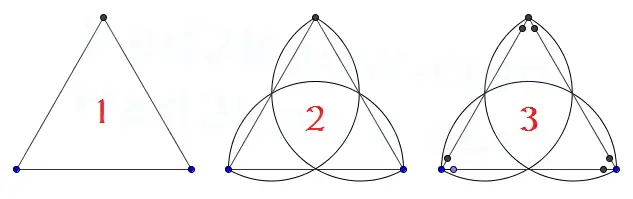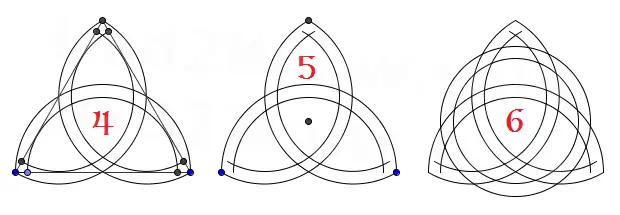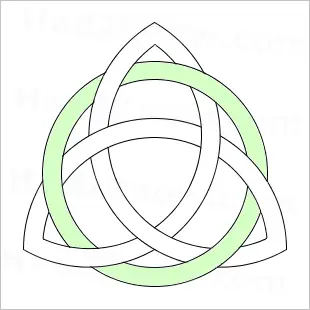# How to Draw a Triquetra/Trinity Knot

The Triquetra symbol, also known as the Trinity Knot, is an ancient symbol that is frequently used as a motif in Celtic knot designs.

In Pagan faiths, the Triquetra may represent things that are threefold, such as air/earth/water or mind/body/soul. In Christianity, it may be used to represent the Holy Trinity. The Trinity Knot is often interlaced with a circle that signifies the unity of the three elements, and in Wicca this symbol is used as protection.

You can draw a Triquetra using circular arcs arranged in an equilateral triangle formation following the steps below.

#### Tools

Graphics software or pencil, ruler, protractor, and compass.

#### Instructions

Step 1: Draw an equilateral triangle, i.e. a triangle in which the angles are all equal to 60°. (See Figure 1 below.)

Step 2: Use the vertices of the triangle as endpoints for three semi-circular arcs. To draw a half-circle over an edge of the triangle, take the midpoint of the edge as the center, and half the length of the edge as the radius. (See Figure 2 below.)

Step 3: After drawing the arcs, mark two points on each edge so that they are short distance from the vertices. (See Figure 3 below.)Step 4: Use the points you drew in Step 3 as the endpoints of three more semi-circular arcs, this time with a slightly smaller radius so that the loops of the knot have some thickness. (See Figure 4 below.)

Step 5: Mark the center of the figure. (See Figure 5 below.)

Step 6: Use the mark you made in Step 5 as the center of two circles, one with a slightly smaller radius than the other. Draw the circles so that they interlace the three loops of the Triquetra. (See Figure 6 below.)Step 7: Finally, erase some of the overlapping segments of the knot and circle to give the figure the appearance of being interlaced.